# (2 + epsilon)-flow conjecture (Solved)

 Importance: High ✭✭✭
 Author(s): Goddyn, Luis A. Seymour, Paul D.
 Subject: Graph Theory » Coloring » » Nowhere-zero flows
 Keywords: edge-connectivity flow
 Recomm. for undergrads: no
 Prize: none
 Posted by: mdevos on: March 7th, 2007
 Solved by: L. M. Lovász, C. Thomassen, Y. Wu, and C.-Q. Zhang. Nowhere-zero 3-flows and modulo k-orientations. J. Combin. Theory Ser. B, 103(5):587–598, 2013.
Conjecture   For everythere exists an integerso that every-edge-connected graph has a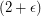-flow.

Definition: Ifis a directed graph and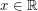, a real-flow (sometimes abbreviated-flow) ofis a real valued flow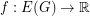with the property thatfor every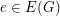. As in the case of nowhere-zero flows, the existence of an-flow in a graph does not depend on the orientation, and we say that an undirected graph has an-flow if some (and thus every) orientation of it admits such a flow. It is a simple exercise to prove that every graph with a real-flow has an (integer) nowhere-zero-flow where. Thus, we may view-flows as a refinement of nowhere-zero-flows.

This conjecture has a strong intuitive appeal. Simply put, the above conjecture asserts that in a graph with high edge-connectivity, it is possible to find a real-valued flow where every edge has about the same flow value. By the above comment relating-flows and nowhere-zero-flows, this conjecture (if true) would imply The weak 3-flow conjecture (See 3-flow conjecture). Zhang [Z] has proved that this result holds when restricted to graphs on a fixed surface, but little else seems to be known.

L. M. Lovász, C. Thomassen, Y. Wu, and C.-Q. Zhang solved this conjecture by proving the following.

For every positive integer, every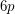-edge-conneected graph admits a nowhere-zero circular-flow.

## Bibliography

[KZ] W. Klostermeyer and C. Q. Zhang,-coloring of planar graphs with large odd-girth. Graph Theory 33 (2000), no. 2, 109--119. MathSciNet

[Z] C. Q. Zhang, Cun Quan, Circular flows of nearly Eulerian graphs and vertex-splitting. J. Graph Theory 40 (2002), no. 3, 147--161. MathSciNet

[LTWZ] L. M. Lovász, C. Thomassen, Y. Wu, and C.-Q. Zhang. Nowhere-zero 3-flows and modulo k-orientations. J. Combin. Theory Ser. B, 103(5):587–598, 2013.

* indicates original appearance(s) of problem.

### $(2+\epsilon)$-flow conjecture.

The-flow conjecture is a consequence of the weak circular flow conjecture which was proved in

[T] C.Thomassen, The weak 3-flow conjecture and the weak circular flow conjecture, J.Combinatorial Theory B 102 (2012) 521-529.

The paper [LTWZ] gives an improved bound.

## Comment viewing options

Select your preferred way to display the comments and click "Save settings" to activate your changes.